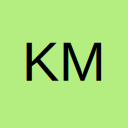# VBA Volitale function controlling rowheight

• Currently the Code works, but only if I enter the data manually into a cell. Currently the cell updates via a formula
I have tried making the application volatile and aplication calculation automatic but it added no difference.

Formula is placed continuously in column H is as follows.
=IF(N7=0,"decrease","expand")

VBA Code is as follows

As stated before currently the code only works if I manually type "decrease" or "expand" into the cells, not when the formula updates automatically.

• Re: VBA Volitale function controlling rowheight

I'm not understanding.
What are you trying to do? What range does cells(target.row,8) refer to?

What does:
Formula is placed continuously in column H is as follows.
=IF(N7=0,"decrease","expand")
mean? Is it the same N7 for each cell or does it change by row, e.g., N7, N8, N9...

• Re: VBA Volitale function controlling rowheight

The change event is not triggered by formula calculation. You have to use the Calculate event but note that it does not have a Target parameter.

• Re: VBA Volitale function controlling rowheight

cells(target.row,8) is the command giving the target area for 8 columns over (column H)
Basically the code says if the words "expand" or "decrease" show up in 8 columns over (column H) the row height changes in which row the word is in.

N7 will change by row eg., N7,N8,N9, and will link to the formula = IF(N7=0,"decrease","expand") Currently in Cell H89 but will go down the H column eg.(H89,H90,H91) etc.

It currently works, but only if it is manually put in. It does not auto update when the formula Currently the formula =IF(N7=0,"decrease","expand") is put into any cell in column H.
The row height will change when the formula is initially put in but not after cell N7 changes from 0 to another number or back to 0

Thanks for the response let me know if you need additional information

• Re: VBA Volitale function controlling rowheight

Thanks,

I think I'm on the write path been editing code but cant get it to work.
Any exact code suggestions?

• Re: VBA Volitale function controlling rowheight

Something like this perhaps. I don't understand your If statement as it repeats itself?

## Participate now!

Don’t have an account yet? Register yourself now and be a part of our community!